# 3. Results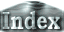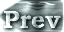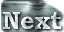### i. What Determines a Runaway Limit? (Description with an Equilibrium Solution of 1D Radiative-Convective Model)

Based on the results shown in previous sections, we will now compare the 1D radiative-convective equilibrium solution and the results of 3D model calculation. It should be noted that relative humidity of the results obtained by 3D numerical calculations is not 100 %. In Nakajima et al. (1992), the all 1D equilibrium solutions assume a value of relative humidity of 100% in the troposphere. Here, we did not attempt to adjust the relative humidity to 100 %, but instead examined the equilibrium solutions in the 1D radiative-convective model with relative humidity fixed at 60 %, which is the value obtained in the 3D calculations. Figure 1 shows the 3D calculation results superimposed on the vertical temperature structure of the 1D equilibrium solution. It can be seen that the temperature structure near the level at which τ = 1 is determined by the moist adiabatic temperature gradient. Furthermore, the upper limit of OLR calculated from the 1D equilibrium solution with relative humidity fixed at 60 % is around 390 W/m2 (Figure 2), and is nearly equivalent to the value of the runaway limit in the 3D model. The upper limit of OLR exists in 1D calculations because the temperature near the τ = 1 level is determined by adiabatic lapse rate and imposes an upper limit in radiation flux at the top of the troposphere. This ultimately implies that the runaway limit in the 3D system is determined by the constraint on radiation flux emitted from the troposphere with considering relative humidity.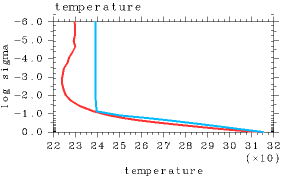Figure 1: The vertical distribution of global mean temperature (red) and that obtained by 1D radiative convective equilibrium model (aqua).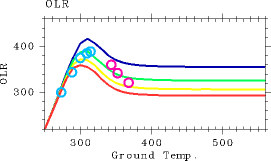Figure 2: The OLR profile (W/m2) as a function of surface temperature (K) obtained by the 1D radiative convective equilibrium model given various relative humidity values: The red line shows 100 %, the yellow line shows 80 %, the green line shows 60 %, and the blue line shows 40 %. Aqua and purple circles show the relationship between global mean surface temperature and OLR calculated by GCM: The aqua circles represent equilibrium states, and the purple circles are in the runaway greenhouse states at Day 1000 or 2000.

Based on the above discussions, it is strongly suggested that an upper limit of outgoing radiation also exist in 3D models based on the equilibrium solution. Therefore, it may be concluded that the present study has successfully established that the thermal runaway state obtained in our 3D numerical calculations corresponds to the runaway greenhouse state , that is, the case where the incident solar radiation flux exceeded the upper limit of outgoing radiation that was determined by equilibrium solutions of Nakajima et al. (1992). Based on this reason, it has also been confirmed that the thermal runaway state obtained for cases of S ≥ 1600 W/m2 can be rightfully referred to as the runaway greenhouse state.

 3.i. What Determines a Runaway Limit? (Description with an Equilibrium Solution of 1D Radiative-Convective Model)